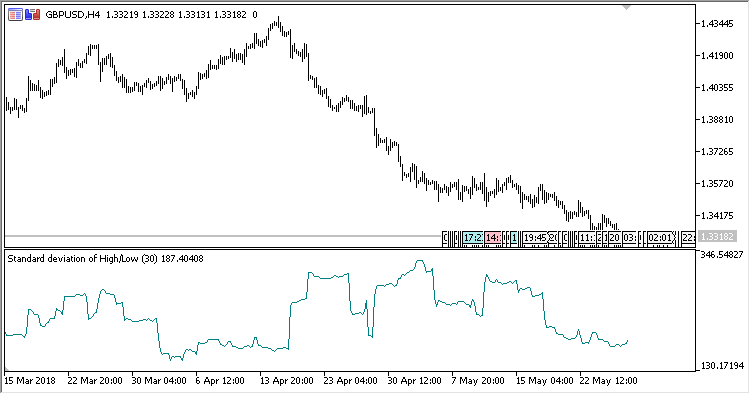# HL_StdDev – indicator for MetaTrader 5

The oscillator shows standard deviation calculated on the difference between the High and the Low.

It has two input parameters:

• Period – standard deviation calculation period;
• Method – standard deviation calculation method.
Related Posts

Calculations:

`HL_StdDev = Standard Deviation (HL)`

where:

`HL = High-Low`